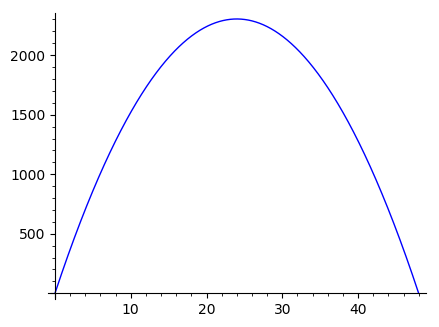# A new software company wants to start selling DVDs with their product. The manager notices that...

## Question:

A new software company wants to start selling DVDs with their product. The manager notices that when the price for a DVD is 15 dollars, the company sells 132 units per week. When the price is 27 dollars, the number of DVDs sold decreases to 84 units per week. Answer the following questions:

A. Assume that the demand curve is linear. Find the demand, q, as a function of price, p.

B. Write the revenue function, as a function of price.

C. Find the price that maximizes revenue. Hint: you may sketch the graph of the revenue function. Round your answer to the closest dollar.

D. Find the maximum revenue, i.e., the revenue that corresponds to your answer for the preceding question.

## Revenue:

The revenue acquired from selling a product is the total amount of money taken in by selling that product. If a company sells {eq}n {/eq} items at a constant price of {eq}p {/eq}, then their revenue from selling those items is {eq}np {/eq}.

Notice that the revenue doesn't take into account the cost of selling those items: it is purely a measure of how much money the items bring in.

A. If {eq}q(p) {/eq} gives the number of units sold per week at a price of {eq}p {/eq}, then we're given that {eq}q(15)=132 {/eq} and {eq}q(27)=84 {/eq}. Under the assumption that {eq}q {/eq} is a linear function of {eq}p {/eq}, we can find the equation for {eq}q(p) {/eq} by using the two-points formula for a line:

{eq}\begin{align*} \frac{q(p)-q(15)}{q(27)-q(15)}&=\frac{p-15}{27-15}\\ \frac{q(p)-132}{84-132}&=\frac{p-15}{27-15}\\ \frac{q(p)-132}{-48}&=\frac{p-15}{12}\\ q(p)-132&=-4(p-15)&&\text{(multiplying both sides by }-48\text{)}\\ &=-4p+60\\ q(p)&=-4p+60+132\\ &=-4p+192 \, . \end{align*} {/eq}

So the demand curve is given by the equation {eq}\boxed{q(p)=-4p+192}\, {/eq}.

B. Let {eq}r(p) {/eq} be the weekly revenue when the price is {eq}p {/eq}. If the price is {eq}p {/eq}, then the number of units sold per week is {eq}q(p)=-4p+192 {/eq}. So the revenue from selling those units will be

{eq}\begin{align*} r(p)&=pq(p)\\ &=p(-4p+192)\\ &=-4p^2+192p \, . \end{align*} {/eq}

In summary, the revenue function is {eq}\boxed{r(p)=-4p^2+192p}\, {/eq}.

C. The revenue function is plotted below:To find the maximum revenue, we differentiate {eq}r(p)=-4p^2+192p {/eq} with respect to {eq}p {/eq}:

{eq}\begin{align*} r'(p)&=-8p+192\\ &=-8(p-24) \, . \end{align*} {/eq}

So {eq}r'(p)=0 {/eq} only when {eq}p=24 {/eq}. As you can see from the graph (or by noticing that {eq}r(p) {/eq} is a quadratic function with negative leading coefficient), the critical point of {eq}r(p) {/eq} is a maximum. So revenue is maximized when {eq}\boxed{p=24} {/eq}.

D. When {eq}p=24 {/eq}, the revenue is:

{eq}\begin{align*} r(p)&=-4(24^2)+192(24)\\ &=-2304+4608\\ &=2304 \, . \end{align*} {/eq}

So the maximum possible revenue is {eq}\boxed{\\$2304\text{ per week}}\, {/eq}.